×

×

UNF / OTHER / STA 201910STA2014 / What do you call the numerical summary of population?

# What do you call the numerical summary of population? Description

##### Description: This is all of the material we will be covering for Thursday's final. It's mainly just supplementary notes filled with certain definitions and instructions on how to do things.
19 Pages 38 Views 3 Unlocks
Reviews

Elementary Statistics Study Guide—Chapters 1 to 4  (This Is A Basic Summery-An Overview- of Everything You Need to Know):

## What do you call the numerical summary of population?Statistics: The science of collecting, summarizing, organizing,  and analyzing information to draw conclusions and to answer  questions. It’s about providing a measure of confidence in  conclusions. (Numerical summery of a sample.)

There are two types of statistics:

1. Inferential Statistics: This uses methods that generalize  results obtained from a sample to the population. This will

measure the results and see if it is trustworthy.

2. Descriptive Statistics: This will organize and summarize  information that is collected.

Parameter: This is the numerical summery of a population.

Frequency: This is the number of times each category has  occurred.

## What makes histogram more accurate than bar graph?Relative Frequency: This is the percent of observations in a  category. The relative frequency should always add up to one  (100.)

You find the relative frequency by:

1. Dividing the original frequency by the number of  observations in the data (the number of frequencies you add together.) If you want to learn more check out What is another term for volcanic dome?

Frequency/ # of data observations.

2. Next, multiply the decimal/ whatever number you get by

100%. This will give you your relative frequency.

3. Round only if you’re asked to.

Variables: characteristics of individuals in a population. There are two types of variables: Don't forget about the age old question of How is trigonal planar shaped?

1. Qualitative (observational only)

2. Quantitative (measured by numerical data.)

## How does scatter plot illustrate linear regression?Quantitative variables can further be separated into Continuous and Discrete data/ variables:

1. Continuous Data: Can be ANY value in a specified and  relevant interval of values. There are no gaps in this.

Examples of this would be height or weigh.

2. Discrete Data: There are gaps or interruptions within  the values given. Examples would be teeth per child in an  elementary school or salaries.

There are two types of studies: Observational and   Designed

Observational studies are when you simply observe a study  without altering it to obtain a certain outcome. (Just listen and  observe.)

Designed study: These studies are designed and are usually  altered in order to study different outcomes that occur. (A literal  experiment.)Don't forget about the age old question of Are flashbulb memories detailed?

Both of these studies have an explanatory (the independent)  variable and a response (dependent) variable.

More Terms You Need to Know:

1. Sample Mean: Known as this sign x̅

2. Sample Population (population parameter): Known as this sign μ 3. Range The difference between the greatest and smallest values. 4. Midrange The sum of the maximum and the minimum divided by 2

(max+min)/2 We also discuss several other topics like What is an example of vestigial organs?

5. Right Skewed This is when the mean is greater than the median.

(Use the median.)

6. Left Skewed This is when the mean is less than the median. Use

the median.)

7. Symmetrical This is when the mean is equal to the median. (You

can use the mean, standard deviation, or the variance.)

8. Mean This is the average. (Simply add all of your values  together and divide the sum by the amount of values you have.) It is used as a measure of location when there aren’t any outliers. The

distribution shape is symmetric. (Quantitative data.)

9. Median This measures the location when there are extreme outliers.

The distribution shape will be skewed. (Quantitative data.) 10. Mode This measures your location when there is qualitative

data. (Look at which number, letter, or word occurs the most.) 11. IQR Q3­Q1 (Subtract the upper fence from the lower fence.)

This is going to be the range of the middle 50 percent of data. 12. Outliers Anything that is outside the upper and lower fence. It

will NOT be defined by the five­number summery.If you want to learn more check out What is the purpose of tag questions?

13. Lower Fence  To find the lower fence, you have to calculate

Q1­1.5(The IQR).

14. Upper Fence Calculate Q3+1.5(IQR).

15. The Five Number Summery This is all the data that goes onto  a box plot (One of the graphs that you have to know for the test.) The  data that has to be found is the minimum value, Q1, the median (also  known as Q2), Q3, and the maximum. You have to find the five

number summery by placing all given values into the list on your  calculator (EDIT STAT.) Afterwards, you have to go to calc. (STAT CALC 1: 1­Varience Stats.) The calculations for the 5 numbers

should be here.

16. Boxplot A Graphical representation for quantitative data. The

five­number summery is the data you’re using to fill out this graph. 17. No outliers This is when the data is inside the upper and lower

fence. It WILL be defined by the five­number summery. 18. Empirical Rule (a.k.a the 68, 95, and 99.7% rule) A quick

estimate of the spread of data given in a normal distribution when the  mean and the standard deviation is given to you. The data must be  Don't forget about the age old question of What kind of condition is learned helplessness?

symmetric for you to apply this rule.

19. μ ­ = 68%  σ Subtract the sample population from the

variance/standard deviation.

20. μ ­2 =95%  σ Subtract the sample population from 2(the

variance/standard deviation.)

21. μ ­3 =99.7%  σ Subtract the sample population from 3(the  variance/standard deviation.)

22.        R= The Correlation Coefficient. *Remember, if R is close to  ­1, there will be a very weak, negative, linear relationship. If it

close to +1, there will be a positive, strong, linear relationship.* 23. Linear Regression When an independent variable is used to

determine the dependent variable. The difference between the two  will be the independent variable. The independent variable= X. The

dependent variable=Y

24. Linear Regression Equation Ŷ=ax+b       a= the slope.      Y=the y­intercept.      Ŷ= this is just defined as, “y­hat.”    X=the x value (the unknown part of the equation.)

To find this equation on the calculator, put your values into Lists 1 and 2.

1st list= independent variables. 2nd list= dependent variables. (STAT EDIT.) Next, go to calc. (STAT CALC 4: LinReg (ax+b))

If you can’t find the values, go to (2nd press the catalogue button go to DIAGNOSTIC ON) You should find the values afterwards.

Graphs You Need to Know:

1. Bar graph Graphical representation of different data using  bars of different heights. DO NOT LET THEM TOUCH. (They

sometimes have standard error bars.)

2. Histogram This is a more accurate representation for the  distribution of quantitative data. LET THEM TOUCH.

Symmetric (Uniform) shape= use the mean, standard  deviation, or the variance. Which one you use does not

matter.

Left or Right Skewed Shape (with outliers) = Use the

median.

3. Pie Chart Basically, it’s just a circle that’s cut into differently  sized groups depending on the percentage of subjects that are  in those certain groups. Areas of each group (the sectors) will

be proportional to the frequency of the category.

4. Bell Curve (for z scores, etc.) This is known as a normal

distribution.

μ-3σ=0.15%

μ-2σ=2.35%

μ-σ=13.5%

μ=34/34% (This is in the center of the bell curve.)

μ+ σ=13.5%

μ+2 σ=2.35%

μ+3 σ+0.15%

(Learn how to place all of these on the bell curve.) 5. Scatter Plot (for linear regression.) This is a graph where the

values of two variables are plotted on two axes (the x and y  axis). The pattern of the resulting points will reveal if a

correlation is present. It is important to use the Linear  Regression Formula (written above) to find this data.

*Make sure all X and Y axes on every graph is labeled and that you ALWAYS have a title. *

Study Guides Chapter 5 (part 2), Chapter 6, and Chapter 7 A BASIC OVERVIEW OF EVERYTHING THAT WILL BE ON THE TEST. Chapter 5 (part 2.):

Independent events: This is when the occurrence of an event (for example, E)  does not affect the probability of another event (event F).

Dependent Events: The occurrences of an event do affect the probability of event  F in a probability experiment.

Multiplication Rule: If E and F are independent events P(E and F)= P ( E) * P (F )

Multiplication Rule for n Independent Event: If events E1, E2, E3….En, then P(E1 and E2 and E3 and….and En) = P(E1 )* P(E2 )*….* P(En )

Conditional Probability: Notation P(F/E) is, “the probability of event F given event E.” So, this is the probability that event F will occur when given event E in a  probability experiment.

Conditional Probability Rule: If event E and F are any two events, then P(E/F) =  P (E and F) ÷ P (E)= N (E and F) ÷N (E)

This means that the probability of event F happening, when given the occurrence of  E, is found by dividing the probability of E and F by the probability of E. You can also divide the # of outcomes inside of E and F by dividing outcomes of E.

The next two chapters talk about discrete probability distribution and normal probability distribution:

Chapter 6:

Random variable: This is a numerical measure of an outcome within a  probability experiment. It will be determined by chance. They are usually  shown by using the letter X. There are two types of random variables to know  about: discrete variables and continuous variables.

Discrete Random Variables: These are variables that have finite or countable  numbers as their values. For example, it could be number of coin flips or how many  books somebody published

Continuous Random Variables: These are variables that have an infinite amount  of values. Examples would be the speed of a car or a group of peoples’ heights.

Probability Distributions: For a discrete random variable X, it will provide  the random variable and the matching probabilities. It can be a table, graph,  or a formula. Examples below: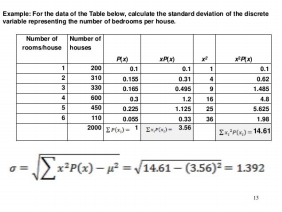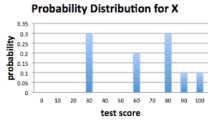Rules for Discrete Probability Distribution: P(X) has to denote the  probability that random variable X will equal x

1. ∑ P(X)=1

2. 0≤P(x)≤1

The Mean of Discrete Random Variable:

This formula gives the mean of a discrete variable.

µx = ∑[x*P(x)]

X will represent the random variable while P(x) will be the probability from  observing the value x.

Binomial Problem Distribution: This is a discrete probability distribution  that describes probabilities of experiments when they have two mutually  exclusive outcomes. These outcomes are referred to as either a failure or a  success.

There are Four Criteria for a Binomial Experiment:

1. First, you will have to check that the experiment is performed a fixed  amount of times. Every representation of an experiment is a trial. 2. Every trial HAS TO BE INDEPENDENT.

3. There has to be TWO mutually exclusive (disjoint) outcomes. These are the successes and the failures.

4. Lastly, know that the probability of success has to be constant  throughout the trials.

Notation Used in the Binomial Probability Distribution:

1. There “n” inside of an independent trial of the experiment. 2. p will denote the probability for each trial. 1-p will represent the  probability of failure for the trials.

3. X will denote the number of success in n independent trials of the  experiment. Therefore, 0 ≤ x ≤ n.

Mean (or Expected Value) and Standard Deviation of a Binomial  Random Variable:

µx= np and x = the square root of np(1-p)

Chapter 7:

Probability Density Function (pdf): This is an equation (that you should  use in your calculator) to help compute the probabilities of continuous  random variables. This function has two rules:

1. The total area under the graph of the equation for all possible  values of the random variable has to equal 1.

2. The graph’s height of the equation has to be greater than or  equal to zero for all possible values of the random variable.

Model: In math, a model is a graph, or equation, or a table. They are used to describe reality. Continuous variables will have to use a model called a  normal curve. This is when the variables are normally distributed. The  histogram will form this shape:*Note: Go over the Empirical Rule again. You will probably need to  know the concept of that for normal distribution problems. *

Standardizing a Normal Random Variable:

Z= x-µ ÷  Know this formula.

Here, the random variable is normally distributed with a mean of 0 and a  standard deviation of 1. Z is considered to be the standard normal  distribution.

Z When you see this sign, just be sure to subtract whatever is in the alpha  from 100%. For example, if you had Z30, you would subtract 100-30 to get 70.

Normal Probability Plot: Graph that plots observed data verses normal  scores (expected Z score value). Example below: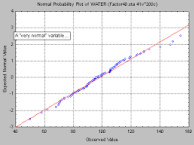Remember, the scatter plots have to be in ascending order, on the line, or within the bands (just pretend

they’re there) in order to conclude the data came from normally  distributed data.

Calculations:

When trying to find binomial/ normal pdf or cdf, just remember to  click 2nd, vars, and then scroll down to find whatever you need. Then just put in your data and you’ll get the answer. The same replies to  INVNORM.

Study Guide: Chapters 5, 6, and 7

1 Out of Three Probability Chapter 5­ Part 1 (The rest of the chapters will be coming out later today.)

*Note: This is an overview of everything you will need to go over for Tuesday’s statistics test. It just gives definitions as well as formulas you have to know. It will not show how to compute certain problems. *

Probability: measurement of the likelihood of a random phenomenon/chance  occurring.

Experiment: Any process with uncertain results that can be repeated.

Sample Space: This is a collection of possible outcomes in a probability  experiment. Example: S {1,2,3,4,5,6}

Event: Any collection of possible outcomes within a probability experiment.

Mutually Exclusive: This is when two events have no outcomes in common. It is  another name for disjoint events.

Probability Model: A list of possible outcomes of a probability experiment as  well as the outcome of each probability. Example: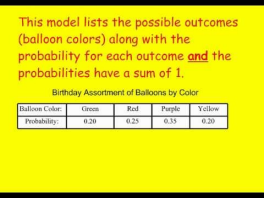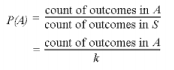*Keep in mind: It is important for you to  understand how to use/ operate a probability model for certain problems.  Study this a lot. *

There are two rules of probability:

1. It is not possible to have probabilities that are less than 0 or greater than 1.  Example: 0 ≤X ≤1.    When the probability of an event is 0, the event is  called impossible.

2. The sum of all probabilities must be equal to 1. This is why when a  probability of n event is equal to 1, it is called certainty.

An Unusual Event: This is what events that have probabilities less than 5% (0.05) are referred to. For example, if you have a probability that is 4%, it is  unusual.

Empirical Method: P(E) = relative frequency of E= frequency of E (divided by)  the number of trials in an experiment.    *This gives the approximate probability of  an event. *

Classical Method: P (E)= # of ways E can occur (divided by) the number of  possible outcomes= m (divided by) n.

*This method of computing probabilities will need equally likely outcomes  (experiments where every outcome has the same probability.)

Tree Diagrams: Used to determine a sample space by listing the equally likely  outcomes of an experiment. Example:Addition Rule for Disjoint (Mutually Exclusive) Events:

P (E and F) = P (E) + P(F)

This means that for any two events, P (E or F) = P (E) + P (F) – P (E and F)

Compliment Rule:

If E will represent any event and Ec represents the compliment of E, then this  means that    P (Ec) = 1= P(E)

∙ Compliment of An Event: Ecis every outcome in the sample space S that ARE NOT outcomes in event E.

For example: P (E or Ec) = P (E) + P (Ec) = P (S) = 1

This means that the event will either happen, or it won’t happen. You will  have to use the Complimentary Rule when you know the probability of an  event will occur, but don’t know the probability of it NOT occurring.

Chapter 8, Chapter 9, and Chapter 10:

∙ Chapter 8 will be on Sampling Distributions (specifically of the sample  mean.)

∙ Chapter 9 will be on Estimation

∙ Chapter 10 will be on Hypothesis Testing.

Chapter 8:

Part One Distribution of the Sample Mean:

Sampling distribution of statistics= a probability distribution for all possible  values of statistics. It’s computed from a sample size of n.

Sampling Distribution of the Sample mean= x-bar (x) is the probability distribution  ̄ of all possible values of the random variable x­bar. This will be computed from a sample size of  n from a population with a mean mu (μ) and standard deviation (sigma) .

Mean of standard deviation of the sampling distribution of x-bar: The  sampling distribution of x bar has a mean

Mu and x bar= standard deviation.

Sigma and x bar= sigma divided by the square root of n. The standard deviation  of the sampling distribution of x bar, sigma x bar, is called the standard error of the  mean.

Two concepts to understand:

1. The mean of the sampling distribution of a sample mean is equal to a mean  of underlying proportion. The standard deviation of a sampling distribution  of a sample mean will be sigma divided by the square root of n (this will  remain despite the sample size.)

2. The Central Limit Theorem: This means that no matter the shape of the  underlying proportion, the sampling distribution of x bar will be  approximately normal as the size, n, increases. It only has to do with x bar.  The more skewed the distribution of a population is, the larger the  sample size needs to be for the Central Limit Theorem.If there is a distribution that isn’t known or isn’t normal, the distribution of the sample mean is about normal. This is only if the sample size is greater  than or equal to 30.

Part Two Distribution of the Sample Proportion:

Sample Proportion: When a random sample of size n is gained from a population  where the individuals may or may not have certain characteristics. It is found by  this formula:

P-hat= x/n

Sampling distribution of p-hat:

∙ The shape of the sampling distribution of p-hat will be approximately normal  is n(1-p) is more than or equal to 5. Sampling size can’t be more than five  percent of the population size.

Chapter 9:

Point Estimate: The value of a statistic that guesses the value of a parameter. It’s  found using p-hat= x/n

Confidence Interval: unknown parameter consisting of an interval of numbers  based on point estimate.

The primary goal of this is to acquire the upper bound and lower bound. Margin of error: This will be p-hat= the upper bound + the lower bound/ 2.

To find all of this, use 1-PropZInt on your calculator.

For T value, you’d plug Stat and Test into your calculator. Scroll down to  number eight and you should find the TInterval.

Example: For mean, if n is greater than or equal to 30, use the t test. If the data  comes from a population approximately normal with no outliers, then use the t interval.

If population standard distribution is known, STAT TEST Option 7 Z interval.

If population standard distribution is now known, use STAT TEST Option 8 T  interval.

Chapter 10 Hypothesis Testing:

Ho This is the null hypothesis. It is shown by an equal sign, greater than or  equal to, or less than or equal to.

Ha This is the alternative hypothesis. This will be the opposite of the signs  above. Examples would be less than, more than, or not equal to.

There are five conditions to hypothesis testing:

1. State the null and the alternative hypothesis.

2. Find the significance level. (α)

3. Determine if it is a random sample. Then determine if you will use  the z or t test. See if n is greater than 30. If it is, continue the test. If sigma is known, use Z test. If sigma is not known, use the T test.  Remember, if the problem says population standard deviation or

normally distributed standard deviation, then sigma will be known. 4. Once you plug the information into your calculator, find the number

of, “p”.

5. See if you have to reject or fail to reject (accept) the null. If P is  greater than the significance level, you will fail to reject (accept) the

null. If P is less than the significance level, reject the null. 6. Finally, give your statement for the problem.

∙ If asked to find the mean for the hypothesis, use mu Ho or Ha (μ)=  whatever number you put. If asked for proportions, use Ho or Ha  (P)= whatever number you put.

Type 1 error: The null hypothesis is true, but it’s rejected.

Type 2 error: The null hypothesis is not true, but it’s accepted anyways.

∙ If the test is 1 tailed, it’s either to the right or the left side. ∙ A two-sided test will only apply when there’s a not equal to (≠) side

Page ExpiredIt looks like your free minutes have expired! Lucky for you we have all the content you need, just sign up here